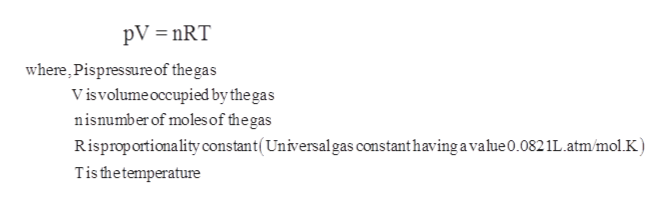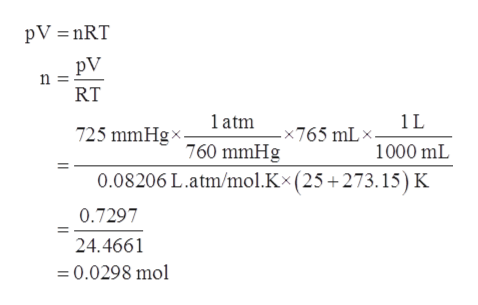# A 1.20 g sample of dry ice is added to a 765 mL flask containing nitrogen gas at a temperature of 25.0 ∘C and a pressure of 725 mmHg . The dry ice is allowed to sublime (convert from solid to gas) and the mixture returns to 25.0 ∘C.What is the total pressure in the flask?

Question
6 views

A 1.20 g sample of dry ice is added to a 765 mL flask containing nitrogen gas at a temperature of 25.0 ∘C and a pressure of 725 mmHg . The dry ice is allowed to sublime (convert from solid to gas) and the mixture returns to 25.0 ∘C.

What is the total pressure in the flask?

check_circle

Step 1

Given information:

Mass of dry ice = 1.20 g

Volume of flask  = 765 mL

Pressure = 725 mmHg

Temperature = 25 ˚C

Step 2

Ideal gas law gives the empirical relationship between pressure, volume, temperature, and amount of gas that is number of moles of a gas. The ideal gas equation is represented as follows:help_outlineImage TranscriptionclosepV nRT where, Pispres sure of the gas Visvolumeoccupied by the gas nisnumber of moles of the gas Risprop ortionality constant(Universalgas constant having a vahue 0.082 1L . atm/mol.K Tis thetemperature fullscreen
Step 3

First number of moles of nitrogen gas are calcul...help_outlineImage TranscriptionclosepV nRT pV n = RT atm 1L 725 mmHgx -x765 mL» 760 mmHg 1000 mL 0.08206 L.atm/mol.Kx (25 +273. 15) K 0.7297 24.4661 0.0298 mol fullscreen

### Want to see the full answer?

See Solution

#### Want to see this answer and more?

Solutions are written by subject experts who are available 24/7. Questions are typically answered within 1 hour.*

See Solution
*Response times may vary by subject and question.
Tagged in

### Chemistry